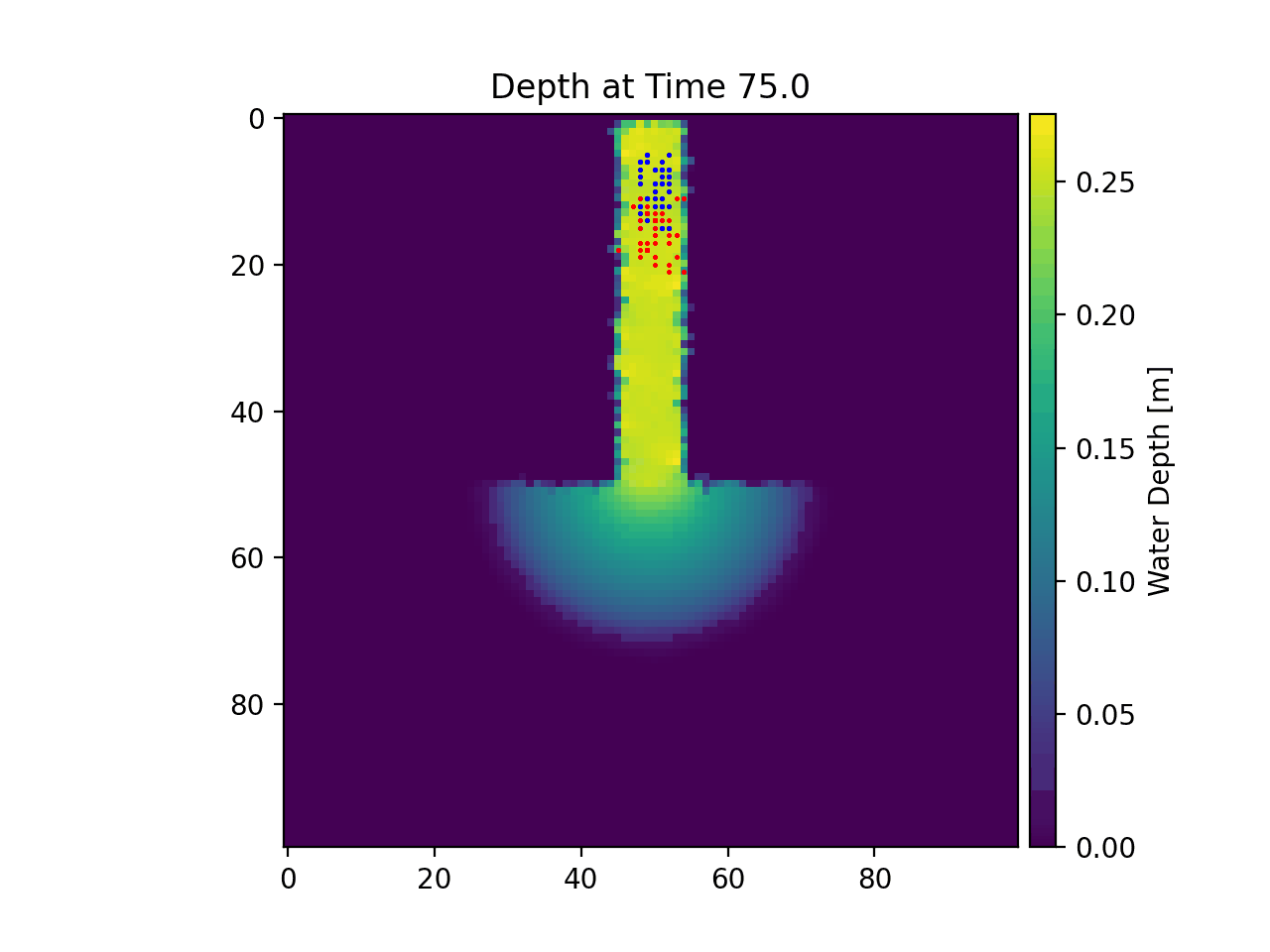# Example 12 - Unsteady Flow Fields¶

In this example we will revisit the ANUGA model domain we looked at in Example 1 - Particles in Steady Flow (ANUGA). This time, however, we will be routing our particles in an unsteady flow field, using the data contained in the examples/example_data subdirectory.

Note

This example must be run from the “examples” directory.

Full example script available `here`.

First we have to load our modules and define the parameter items that are related to the cell size and the particle attributes (number, initial location).

```>>> from dorado.routines import unsteady_plots

>>> # define information that is not in the saved data
>>> dx = 5.
>>> Np_tracer = 50
>>> seed_xloc = list(range(5, 16))
>>> seed_yloc = list(range(48, 53))
```

Now that the basic parameters have been established, we will apply the unsteady_plots() function to perform particle routing over unsteady flow data. The target travel time per timestep is set as 75 seconds.

```>>> walk_data = unsteady_plots(dx, Np_tracer, seed_xloc, seed_yloc,